Custom SearchDIVISION OF A POLYNOMIAL BY A MONOMIAL Division, like multiplication, may be distributive. Consider, for example, the problem (4 + 6 - 2) 2, which may be solved by adding the numbers within the parentheses and then dividing the total by 2. Thus,Now notice that the problem may also-be solved distributively.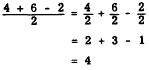CAUTION: Do not confuse problems of the type just described with another type which is similar in appearance but not in final result. For example, in a problem such as 2 (4 + 6 - 2) the beginner is tempted to divide 2 successively by 4, then 6, and then -2, as follows: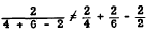Notice that we have canceled the "equals" sign, because 2 + 8 is obviously not equal to 1/2 + 2/6. - 1. The distributive method applies only in those cases in which several different numerators are to be used with the same denominator When literal numbers are present in an expression, the distributive method must be used, as in the following two problems:Quite often this division may be done mentally, and the intermediate steps need not be written out. DIVISION OF A POLYNOMIAL BY A POLYNOMIAL Division of one polynomial by another proceeds as follows: 1. Arrange both the dividend and the divisor in either descending or ascending powers of the same letter. 2. Divide the first term of the dividend by the first term of the divisor and write. the result as the first term of the quotient. 3. Multiply the complete divisor by the quotient just obtained, write the terms of the product under the like terms of the dividend, and subtract this expression from the dividend. 4. Consider the remainder as a new dividend and repeat steps 1, 2, and 3. EXAMPLE: (10x3 - 7x2y - 16xyz2 + 12y3) + (5x - 6y) SOLUTION: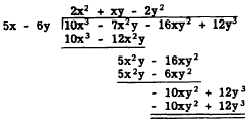In the example just shown, we began by dividing the first term, 10x3, of the dividend by the first term, 5x, of the divisor. The result is 2x2. This is the first term of the quotient. Next, we multiply the divisor by 2x2 and subtract this product from the dividend. Use  the remainder as a new dividend. Get the second term, xy, in the quotient by dividing the first term, 5x2y, of the new dividend by the first term, 5x,of the divisor. Multiply the divisor by xy and again subtract from the dividend.  Continue the process until the remainder is zero or is of a degree lower than the divisor. In the example being considered, the remainder is zero (indicated by the double line at the bottom). The quotient is 2x2 + xy - 2y2. The following long division problem is an example in which a remainder is produced: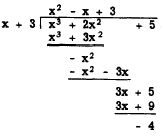The remainder is -4. Notice that the term -3x in the second step of this problem is subtracted from zero, since there is no term containing x in the dividend. When writing down a dividend for long division, leave spaces for missing terms which may enter during the long division process. In arithmetic, division problems are often arranged as follows, in order to emphasize the relationship between the remainder and the divisor :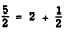This same type of arrangement is used in algebra. For example, in the problem just shown, the results could be written as follows:Remember, before dividing polynomials arrange the terms in the dividend and divisor according to either descending or ascending powers of one of the literal numbers. When only one literal number occurs, the terms are usually arranged in order of descending powers.. For example, in the polynomial 2x2 + 4x3 + 5 - 7x the highest power among the literal terms is x3. If the terms are arranged according to descending powers of x, the term in x3 should appear first. The x3 term should be followed by the x term, the x term, and finally the constant term. The polynomial arranged according to descending powers of x is 4x3 + 2x2 - 7x + 5. Suppose that 4ab + b2 + 15a2 is to be divided by 3a + 2b. Since 3a can be divided evenly into 15a2, arrange the terms according to descending powers of a. The dividend takes the form 15a2 + 4ab + b2Integrated Publishing, Inc. - A (SDVOSB) Service Disabled Veteran Owned Small Business